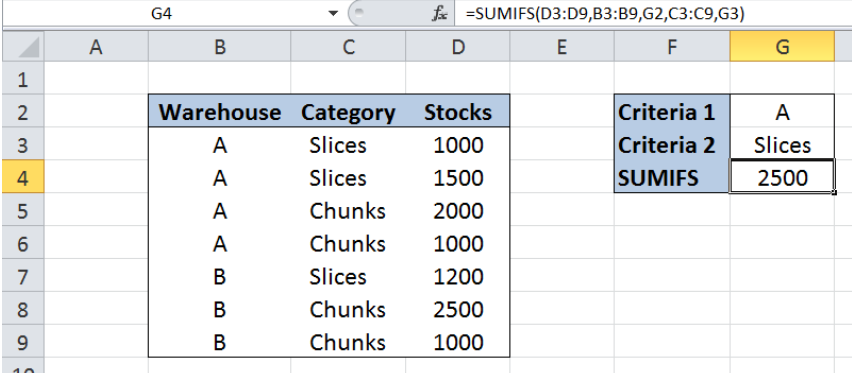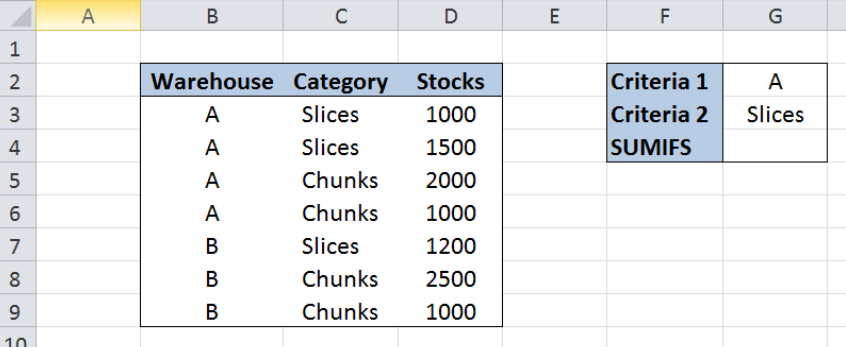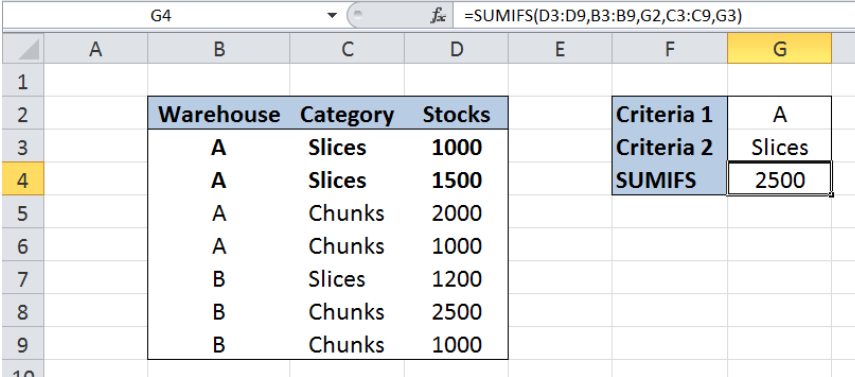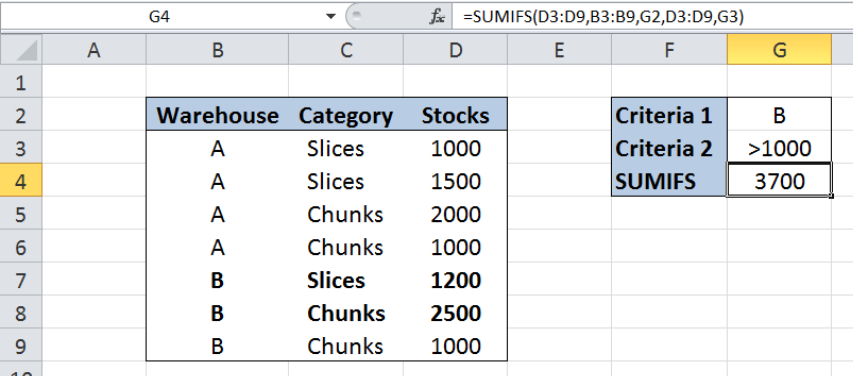Get instant live expert help with Excel or Google Sheets“My Excelchat expert helped me in less than 20 minutes, saving me what would have been 5 hours of work!”

#### Post your problem and you’ll get Expert help in seconds.

Your message must be at least 40 characters
Our professional Expert are available now. Your privacy is guaranteed.

# Excel SUMIFS Function

While working with Excel, we are able to sum values based on one or more criteria by using the SUMIFS function.  This step by step tutorial will assist all levels of Excel users in the usage and syntax of SUMIFS function.Figure 1. Final result: Excel SUMIFS function

Final formula using cell reference in criteria:  `=SUMIFS(D3:D9,B3:B9,G2,C3:C9,G3)`

Final formula using text strings in criteria:  `=SUMIFS(D3:D9,B3:B9,"A",C3:C9,"Slices")`

## Syntax of SUMIFS Function

`=SUMIFS(sum_range, criteria_range1, criteria1, [criteria_range2, criteria2], ...)`

• sum-range – the cells whose values we want to sum based on the given conditions
• criteria_range1: the data range that will be evaluated using criteria1
• criteria1:  the criteria or condition that determines which cells will be added
• criteria_range2 and criteria2 are optional; only applied when there are more than one criteria that needs to be satisfied
• criteria_range should have the same number of rows and columns as sum_range

## Setting up our Data

Our data consists of three columns : Warehouse (column B), Category (column C) and Stocks (column D).  We have specified two criteria in cells G2 and G3: Warehouse “A” and Category “Slices”. We want to calculate the total stocks based on the two given criteria.  The result will be recorded in cell G4.Figure 2. Sample data  to sum values using SUMIFS

## Sum stocks for Warehouse A and Slices

We want to sum the stocks in column D when the corresponding warehouse is “A” and the category is “Slices”.  Let us follow these steps:

Step 1.  Select cell G4

Step 2.  Enter the formula: `=SUMIFS(D3:D9,B3:B9,G2,C3:C9,G3)`

Step 3.  Press ENTERFigure 3.  Sum stocks for Warehouse “A” and Slices using SUMIFS

Our sum_range is D3:D9, which is the column we want to sum.  The first criteria in cell G2 is “A and the range is B3:B9, which contains the values for warehouse.  The second criteria in cell G3 is “Slice” and the range is C3;C9, which contains the values for category.

Our formula only sums the stocks in column D when both criteria are satisfied: Warehouse must be “A” and Category must be “Slices”.  As a result, the value in G4 is 2500, which is the sum of D3 and D4, both satisfying the two criteria we specified.

## Sum stocks >1000 for Warehouse B

This time, we want to sum the stocks in column D when the corresponding warehouse is “B” and the value of stocks is greater than 1000.  Let us follow these steps:

Step 1.  Enter “B” in cell G2 and “>1000” in cell G3

Step 2.  Select cell G4

Step 3.  Enter the formula: `=SUMIFS(D3:D9,B3:B9,G2,D3:D9,G3)`

Step 4.  Press ENTERFigure 4.  Sum stocks greater than 1000  for Warehouse “B” using SUMIFS

Note that our formula is similar to our previous example, but this time, the range for the second criteria is D3:D9, because we want to sum only stocks > 1000.

As a result, the value in G4 is 3700, which is the sum of D7 and D8, both satisfying the two criteria we specified.

## Notes

• Criteria can be inputted directly into our formula but must be enclosed in double quotes; for example, our two formulas can be entered as:
• Example 1: `=SUMIFS(D3:D9,B3:B9,"A",C3:C9,"Slices")`
• Example 2: `=SUMIFS(D3:D9,B3:B9,"B",D3:D9,">1000")`
• We can use the wildcard characters ? and * in our criteria; A question mark means any one character while an asterisk means any sequence of characters
• We can enter up to 127 pairs of criteria and range into a SUMIFS formula
• SUMIFS can handle ranges but not arrays

Most of the time, the problem you will need to solve will be more complex than a simple application of a formula or function. If you want to save hours of research and frustration, try our live Excelchat service! Our Excel Experts are available 24/7 to answer any Excel question you may have. We guarantee a connection within 30 seconds and a customized solution within 20 minutes.

### Did this post not answer your question? Get a solution from connecting with the expert.Another blog reader asked this question today on Excelchat:
Solution examplesI am trying to utilize a complicated sumif or sumifs formula that can pull a total amount from a date and a drop down box from another tab.
Solved by E. B. in 15 minsI have a spreadsheet that totals invoices. A bunch of cells at the bottom of the spreadsheet calculates totals based on which department the invoice is charged to. I'd like to add a function where it also considers the date (so if it's an invoice in January, it goes to a January total, February, etc.). But I can't figure out how to write it properly...
Solved by K. J. in 27 minsIf I want to write a formula that states" "If this name shows up K24, and this word shows up in J24 pull this number from M24" I can creat that formula - however I need to drag this down so it pulls from row 24 - 60 - is there way to do this?
Solved by F. D. in 40 minsamending a formula so that there is another criteria and if it does not meet this criteria then we stick with the original criteria
Solved by A. B. in 40 minsSo im trying to use a sumif formula, but i need it to not look for a range for one specific criteria but instead look for what one specific field says. Example Below =sum(sumifs(QuantityMC,TeamsMC,A6,TypeMC,B2)) The above formula works as on the specific MC sheet an entire column is dedicated for "TypeMC". I want to be able to delete this column and have "TypeMC" to be a single field as it will be the same for every item on that sheet but may differ on a different sheet.
Solved by M. H. in 45 mins## Subscribe to Excelchat.coAnother blog reader asked this question today on Excelchat: